# Löwner method

Jump to: navigation, search

Löwner's method of parametric representation of univalent functions, Löwner's parametric method

A method in the theory of univalent functions that consists in using the Löwner equation to solve extremal problems. The method was proposed by K. Löwner . It is based on the fact that the set of functions,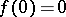, that are regular and univalent in the disc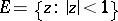and that maponto domains of type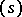(cf. Smirnov domain), which are obtained from the discby making a slit along a part of a Jordan arc starting from a point on the circle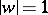and not passing through the point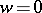, is complete (in the topology of uniform convergence of functions inside) in the whole family of functions,, that are regular and univalent inand are such that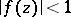in. Associating the length of the arc that has been removed with a parameter, it has been established that a function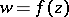,, that mapsunivalently onto a domainof typeis a solution of the differential equation (see Löwner equation)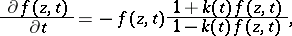(*), satisfying the initial condition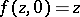. Hereand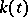is a continuous complex-valued function on the intervalcorresponding towith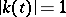. Löwner used this method to obtain sharp estimates of the coefficients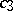and,in the expansions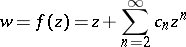and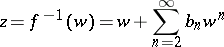in the classof functions,,, that are regular and univalent in.

The Löwner method has been used (see ) to obtain fundamental results in the theory of univalent functions (distortion theorems, reciprocal growth theorems, rotation theorems). Let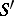be the subclass of functionsinthat have inthe representation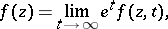where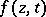, as a function of, is regular and univalent in,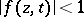in,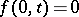,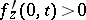, and as a function of,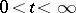, is a solution of the differential equation (*) satisfying the initial condition;in (*) is any complex-valued function that is piecewise continuous and has modulus 1 on the interval. To estimate any quantity on the classit is sufficient to estimate it on the subclass, since any functionof classcan be approximated by functions,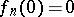,, each of which mapsunivalently onto the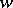-plane with a slit along a Jordan arc starting atand not passing through, and hence by functions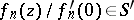. Under this approximation the quantities to be estimated for the approximating functions converge to the same quantity as for the function.

Löwner's method has been used in work on the theory of univalent functions (see ); it often leads to success in obtaining explicit estimates, but as a rule it does not ensure the classification of all extremal functions and does not give complete information about their uniqueness. For a complete solution of extremal problems Löwner's method is usually combined with a variational method (see  and Variation-parametric method). Löwner's method has been extended to doubly-connected domains. A generalized equation of the type of Löwner's equation has been obtained for multiply-connected domains and for automorphic functions (see ).